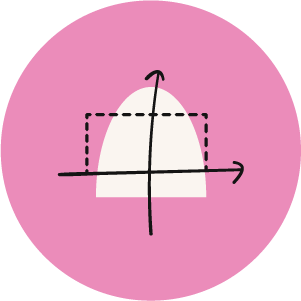Math
IGCSE

## Math M/J 2024 (Hybrid)

#### Haroon Tariq

Teaching 84+ students

I taught 251 students on Noon so far, and I look forward to teaching you.

Group Duration

Started from June 11!

### Curriculum Progress

0/9 chapters complete
Chapter 1Number
ongoing
Numbers & Accuracy
Sets & Venn Diagrams
Conversion
Order by Size
Standard Form
Working with Fraction
Bounds
Ratios
Percentages
Using a Calculator
Time
Currency
Exponential Growth & Decay (Simple and Compound Interest)
Revision
Chapter 2Algebra & graphs
ongoing
Using Algebra
Factorising & Expanding
Algebraic Fractions
Indices
Linear Equations
Simultaneous Linear Equations
Linear Inequalities (no graphs)
Graphical Inequalities (Graphs)
Sequences
Direct & Inverse Proportion
Functions
Speed, Distance & Time
Working with Graphs
Chapter 3Co-ordinate geometry
scheduled
Chapter 4Geometry
scheduled
Chapter 5Mensuration (Perimeters, Areas & Volumes)
scheduled
Chapter 6Trigonometry
scheduled
Chapter 7Vectors and transformations
scheduled
Chapter 8Probability
scheduled
Chapter 9Statistics
scheduled
Monthly|Tax included
One Time Payment|Tax included

We will be completing the syllabus for M/J 2024 session.

Competition
Session
Assignment
exam
Focused on hardest chaptersDedicated Q&A sessions